PRINTABLE FOR KIDS

XII (12) HSC

XI (11) FYJC
X (10) SSC

# Question 1.Mother is 25 years older than her son. Find son’s age if after 8 years ratio of son’s age to mother’s age will be 4/9Answer:let the age of son be x, so age of mother is x + 25After 8 years, gather e of son is x + 8 and age of mother is x + 25 + 8So according to give conditions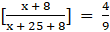Now solving the equationMultiplying both sides by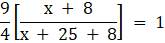Multiplying both sides by 4(x + 25 + 8)9(x + 8) = 4(x + 33)Expanding the equation9x + 72 = 4x + 132Adding – 4x – 72 on both sides5x = 60Dividing by 10 on both sidesx = 12So, age of son is 12 yearsQuestion 2.The denominator of a fraction is greater than its numerator by 12. If the numerator is decreased by 2 and the denominator is increased by 7, the new fraction is equivalent with 1/2. Find the fraction.Answer:let the numerator be x, so dthe enominator is x + 12New numerator is x – 2 , newthe denominator is x + 12 + 7So according to given conditions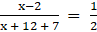Multiplying both sides by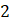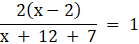Multiplying by x + 19 on both sides2(x – 2) = x + 192x – 4 = x + 19Adding – x on both sidesx – 4 = 19Adding 4 on both sidesx = 23So, numerator is 23 and denominator is 12 + 23 = 35Required fraction is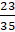Question 3.The ratio of weights of copper and zinc in brass is 13:7. Find the weight of zinc in a brass utensil weighing 700 gm.Answer:let the weight of zinc be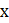copper/zinc = copper/x = 13/7copper = 13x/7So according to given conditionsx + 13x/7 = 700Multiplying both side by 77x + 13x = 490020x = 4900Dividing both side by 20x = 245So, weight of zinc is 245gQuestion 4.Find three consecutive whole numbers whose sum is more than 45 but less than 54.Answer:let the lowest number be, so other numbers are x + 1And x + 2According to given conditions 45 < x + (x + 1) + (x + 2) < 54Solving this equation, we have 45 < 3x + 3 < 54Adding – 3 on both sides42 < 3x < 51Dividing by 3 on both sides14 < x < 17So x = 15 or x = 16So consecutive numbers are 15,16,17 or 16,17,18Question 5.In a two-digit number, a digit at the ten’s place is twice the digit at unit’s place. If the number obtained by interchanging the digits is added to the original number, the sum is 66. Find the numberAnswer:let the number at digit place beSo, da igit at tens place is 2x.Number is 10×2x + x = 21xNow interchanging the digits, at digit place we have 2x and at tens place x.number is 10×x + 2x = 12xAccording to the given condition’s 12x + 21x = 6633x = 66Dividing both side by 33X = 2Digit at tens place is 2 × 2 = 4Number is 42Question 6.Some tickets of Rs.200 and some of Rs.100, of a drama in a theatre were sold. The number of tickets for Rs.200 sold was 20 more than the number of tickets for Rs.100. The total amount received by the theatre by the sale of tickets was Rs.37000. Find the number of Rs.100 tickets sold.Answer:let the number of Rs.100 ticket sold be xNumber of Rs.200 ticket sold is x + 20According to given conditions 100x + 200(x + 20) = 37000300x + 4000 = 37000Adding - 4000 on both sides300x = 33000Dividing both side by 300x = 110Number of Rs.100 tickets sold are 110Question 7.Of the three consecutive natural numbers, five times the smallest number is 9 more than four times the greatest number, find the numbers.Answer:let the numbers be x,x + 1,x + 2 of which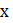being the smallest. According to given conditions 5x = 9 + 4(x + 2)Expanding5x = 9 + 4x + 8Adding - 4x on both sidesx = 17So, numbers are 17,18,19Question 8.Raju sold a bicycle to Amit at 8% profit. Amit repaired it spending Rs.54. Then he sold the bicycle to Nikhil for Rs.1134 with no loss and no profit. Find the cost price of the bicycle for which Raju purchased it.Answer:let the cost of the cycle for Raju be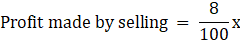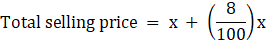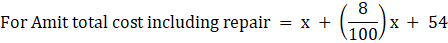So according to given conditions total cost = total selling price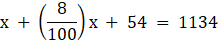Adding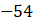on both sides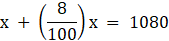Multiplying by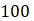on both side100x + 8x = 108000108x = 108000Dividing by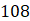on both sidex = 1000So, cost of cycle to Raju is Rs.1000Question 9.A Cricket player scored 180 runs in the first match and 257 runs in the second match. Find the number of runs he should score in the third match so that the average of runs in the three matches be 230.Answer:let the runs required in third match beSo according to given conditions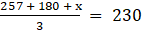Multiplying both side by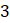257 + 180 + x = 690Subtracting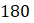from both sides257 + x = 510Subtracting 257 from both sidesx = 253Therefore 253 runs are required.Question 10.Sudhir’s present age is 5 more than three times the age of Viru. Anil’s age is half the age of Sudhir. If the ratio of the sum of Sudhir’s and Viru’s age to three times Anil’s age is 5:6, then find Viru’s age.Answer:let the age of Viru beSudhir age = 3x + 5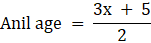According to given conditions,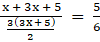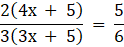Multiplyingon both side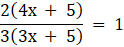Multiplying 3(3x + 5) on both sides2(4x + 5) = 3(3x + 5)Expanding8x + 10 = 9x + 15Adding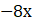on both sideX + 15 = 10Adding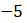on both sidex = – 5But since age cannot be negative so, x = 5Hence age is 5 years.

## PDF FILE TO YOUR EMAIL IMMEDIATELY PURCHASE NOTES & PAPER SOLUTION. @ Rs. 50/- each (GST extra)

SUBJECTS

HINDI ENTIRE PAPER SOLUTION

MARATHI PAPER SOLUTION
SSC MATHS I PAPER SOLUTION
SSC MATHS II PAPER SOLUTION
SSC SCIENCE I PAPER SOLUTION
SSC SCIENCE II PAPER SOLUTION
SSC ENGLISH PAPER SOLUTION
SSC & HSC ENGLISH WRITING SKILL
HSC ACCOUNTS NOTES
HSC OCM NOTES
HSC ECONOMICS NOTES
HSC SECRETARIAL PRACTICE NOTES

2019 Board Paper Solution

HSC ENGLISH SET A 2019 21st February, 2019

HSC ENGLISH SET B 2019 21st February, 2019

HSC ENGLISH SET C 2019 21st February, 2019

HSC ENGLISH SET D 2019 21st February, 2019

SECRETARIAL PRACTICE (S.P) 2019 25th February, 2019

HSC XII PHYSICS 2019 25th February, 2019

CHEMISTRY XII HSC SOLUTION 27th, February, 2019

OCM PAPER SOLUTION 2019 27th, February, 2019

HSC MATHS PAPER SOLUTION COMMERCE, 2nd March, 2019

HSC MATHS PAPER SOLUTION SCIENCE 2nd, March, 2019

SSC ENGLISH STD 10 5TH MARCH, 2019.

HSC XII ACCOUNTS 2019 6th March, 2019

HSC XII BIOLOGY 2019 6TH March, 2019

HSC XII ECONOMICS 9Th March 2019

SSC Maths I March 2019 Solution 10th Standard11th, March, 2019

SSC MATHS II MARCH 2019 SOLUTION 10TH STD.13th March, 2019

SSC SCIENCE I MARCH 2019 SOLUTION 10TH STD. 15th March, 2019.

SSC SCIENCE II MARCH 2019 SOLUTION 10TH STD. 18th March, 2019.

SSC SOCIAL SCIENCE I MARCH 2019 SOLUTION20th March, 2019

SSC SOCIAL SCIENCE II MARCH 2019 SOLUTION, 22nd March, 2019

XII CBSE - BOARD - MARCH - 2019 ENGLISH - QP + SOLUTIONS, 2nd March, 2019

HSC Maharashtra Board Papers 2020

(Std 12th English Medium)

HSC ECONOMICS MARCH 2020

HSC OCM MARCH 2020

HSC ACCOUNTS MARCH 2020

HSC S.P. MARCH 2020

HSC ENGLISH MARCH 2020

HSC HINDI MARCH 2020

HSC MARATHI MARCH 2020

HSC MATHS MARCH 2020

SSC Maharashtra Board Papers 2020

(Std 10th English Medium)

English MARCH 2020

HindI MARCH 2020

Hindi (Composite) MARCH 2020

Marathi MARCH 2020

Mathematics (Paper 1) MARCH 2020

Mathematics (Paper 2) MARCH 2020

Sanskrit MARCH 2020

Important-formula

THANKS